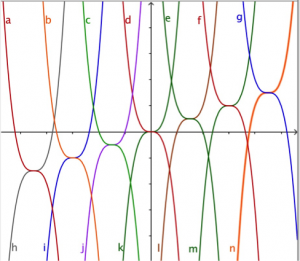The picture shows some curves in the family of y = x cubed. Can you find the graph of this function and the equations of the other graphs? In this workshop guide you will find more examples of this type of ‘inverse problem’ where the learner is given information and has to work ‘backwards’, in this case using their knowledge of functions, graphs and transformations. The formula for solving quadratic equations is derived using transformations of the parabola y = x squared. Graphing software such as Geogebra can be used for these activities but it is preferable to work on them without the software and perhaps use it only for learners to create their own patterns.